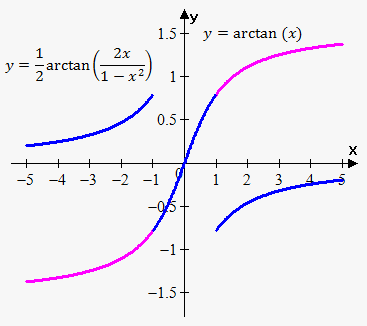# Condition of Constancy of the Function

Fact. Suppose function y=f(x) is defined and continuous on interval X and has finite derivative f'(x). Function y=f(x) is constant if and only if f'(x) for all x in X.

This fact means that if on some interval derivative of function equals 0 then function is constant their, its graph is just horizontal line.

Corollary. Suppose two function y=f(x) and y=g(x) are defined and continuous on interval X, and have finite derivatives f'(x) and g(x). Function y=f(x) is constant if and only if f'(x) for all x in X. If f'(x)=g'(x) on interval X then f(x)=g(x)+C, where C is a constant for all x in X.

This corollary means that if functions have same derivatives on interval X then their difference is constant.

Example 1 . Consider functions f(x)=arctan(x) and g(x)=arcsin(x/(sqrt(1+x^2))).

They are defined and continuous on interval (-oo,oo).

Since f'(x)=1/(1+x^2) and g'(x)=1/(1-(x/sqrt(1+x^2))^2)*(sqrt(1+x^2)-(x^2)/(sqrt(1+x^2)))/(1+x^2)=1/(1+x^2) then according to corollary arctan(x)=arcsin(x/(sqrt(1+x^2)))+C on (-oo,oo).

To find constant plug any value of x, for example, x=0: arctan(0)=arcsin(0/sqrt(1+0^2))+C or C=0.

So, we have the following fact: arctan(x)=arcsin(x/sqrt(1+x^2)) for all x.

Example 2. Consider functions f(x)=arctan(x) and g(x)=1/2 arctan((2x)/(1-x^2)).

It can be easily proven that f'(x)=g'(x). However, function g(x) is not defined when x=+-1. So, 1/2 arctan((2x)/(1-x^2))=arctan(x)+C on (-oo,-1),(-1,1),(1,oo).

It is interesting that constant will be different for different intervals.For interval (-1,1) we plug x=0: 1/2 arctan((2*0)/(1-0^2))=arctan(0)+C or C=0.

For interval (-oo,-1) we let x->-oo: 0=-pi/2+C or C=pi/2.

For interval (1,oo) we let x->oo: 0=pi/2+C or C=-pi/2.

So,

1/2arctan((2x)/(1-x^2))=arctan(x)+pi/2 on (-oo,-1),

1/2arctan((2x)/(1-x^2))=arctan(x) on (-1,1),

1/2arctan((2x)/(1-x^2))=arctan(x)-pi/2 on (1,oo).

Graph confirms these facts.